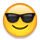# Sumifs formula for children rows if meet a certain criteria (using sheet reference)

Hi,

I am looking for a formula to use in my metric sheet to sum the children rows that meet a certain criteria. I have tried everything I could think of and have had no luck. I have my source sheet with the parent/children rows, and then a separate metric sheet I am using to put the formulas in and referencing my source sheet. Do I need to add some helper rows to my source sheet to do this?

I need to sum the children rows in Column A, if the criteria in the grandchildren rows (column= X

Any help is appreciated.

Thank you,

Crystal

• You will need a helper column or two on the source sheet for this.

I would suggest one that displays the level of hierarchy using a formula such as

=COUNT(ANCESTORS([Column Name]@row))

.

Then you can use that as an additional range/criteria in your metrics sheet.

Parent rows will have a value of 0.

Children = 1

Grandchildren = 2

Great Grandchildren = 3

so on and so forth...

• Hi Paul,

Thank you. I added a helper hierarchy row to the source sheet and used it as an additional range, but still didn't work. I need to sum the numbers in the child row, but the criteria for that sum is in the grandchildren row. Is that why it isn't working?

I need to get the sums of the numbers in column A if the rest of the criteria is met.

sum the totals in column A-child row, if column B- grandchildren row has a value of X

Thank you,

Crystal

Use a helper column on the source sheet with an IF statement in it along the lines of...

=IF(CHILDREN([Column B]@row) = "X", [Column A]@row)

.

If you put this in the parent rows, you can use the IF to determine your criteria and populate the value from Column A if the criteria matches.

.

Then your Metrics sheet would just sum this helper column.